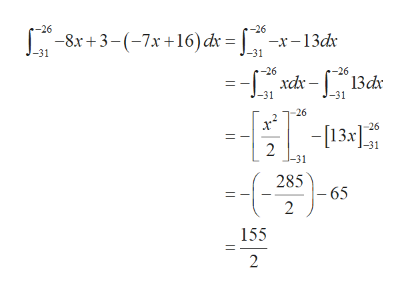# Determine the area, in square units, of the region bounded above by g(x)=−8x+3 and below by f(x)=−7x+16 over the interval [−31,−26]. Do not include any units in your answer.

Question
2 views

Determine the area, in square units, of the region bounded above by g(x)=−8x+3 and below by f(x)=−7x+16 over the interval [−31,−26]. Do not include any units in your answer.

check_circle

Step 1

Given g(x)=−8x+3 and f(x)=−7x+16

And the curve g(x) is bounded above and f(x)=−7x+16 is bounded below over the interval [−31,−26]

Step 2

Therefore, the ...help_outlineImage Transcriptionclose-26 -26 -8x +3-(-7x +16) dr = | —х -13dx -31 -31 =-ná-"Bát c-26 -26 =- xdx – 13dx J-31 J-31 -26 -[13x -26 -31 285 - 65 2 155 2 || fullscreen

### Want to see the full answer?

See Solution

#### Want to see this answer and more?

Solutions are written by subject experts who are available 24/7. Questions are typically answered within 1 hour.*

See Solution
*Response times may vary by subject and question.
Tagged in

### Integration